Hello -

I spent a few hours searching through the knowledge base and trying different formulas but I cant seem to make this work.

Would anyone be willing to point me in the right direction?

I am trying to take this series: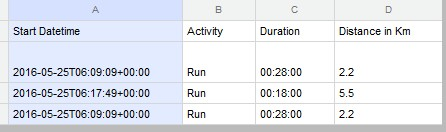and put it into a line chart where the data is SUMMARIZED BY MONTH and not by individual days like the below.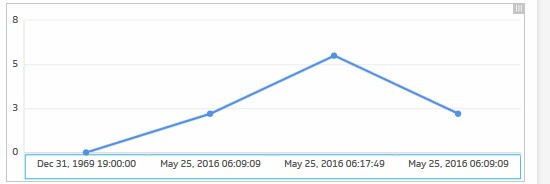Thanks!

•K M Official comment

Hello Keith,

You can meet your requirement by using Klipfolio's DATE_CONVERT, LOOKUP, GROUP, GROUPBY functions.

Here is the logic-

A. For x-axis: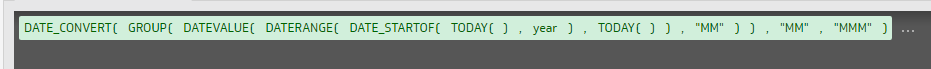This will display all 12 months of the year on your x-axis.

**Note: You can also hard-code the 12 months in here. (ARRAY(Jan, Feb, Mar,.....))

B. For Series: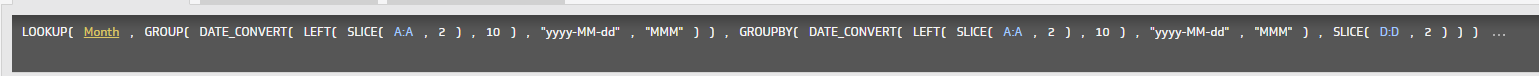This will show the total distance by month.

GROUP function is used to remove duplicates. GROUPBY function is used for grouping the data by months. LOOKUP is used to align data with your x-axis. And DATE_CONVERT is used to get the date in needed format.

Your final klip would look like this-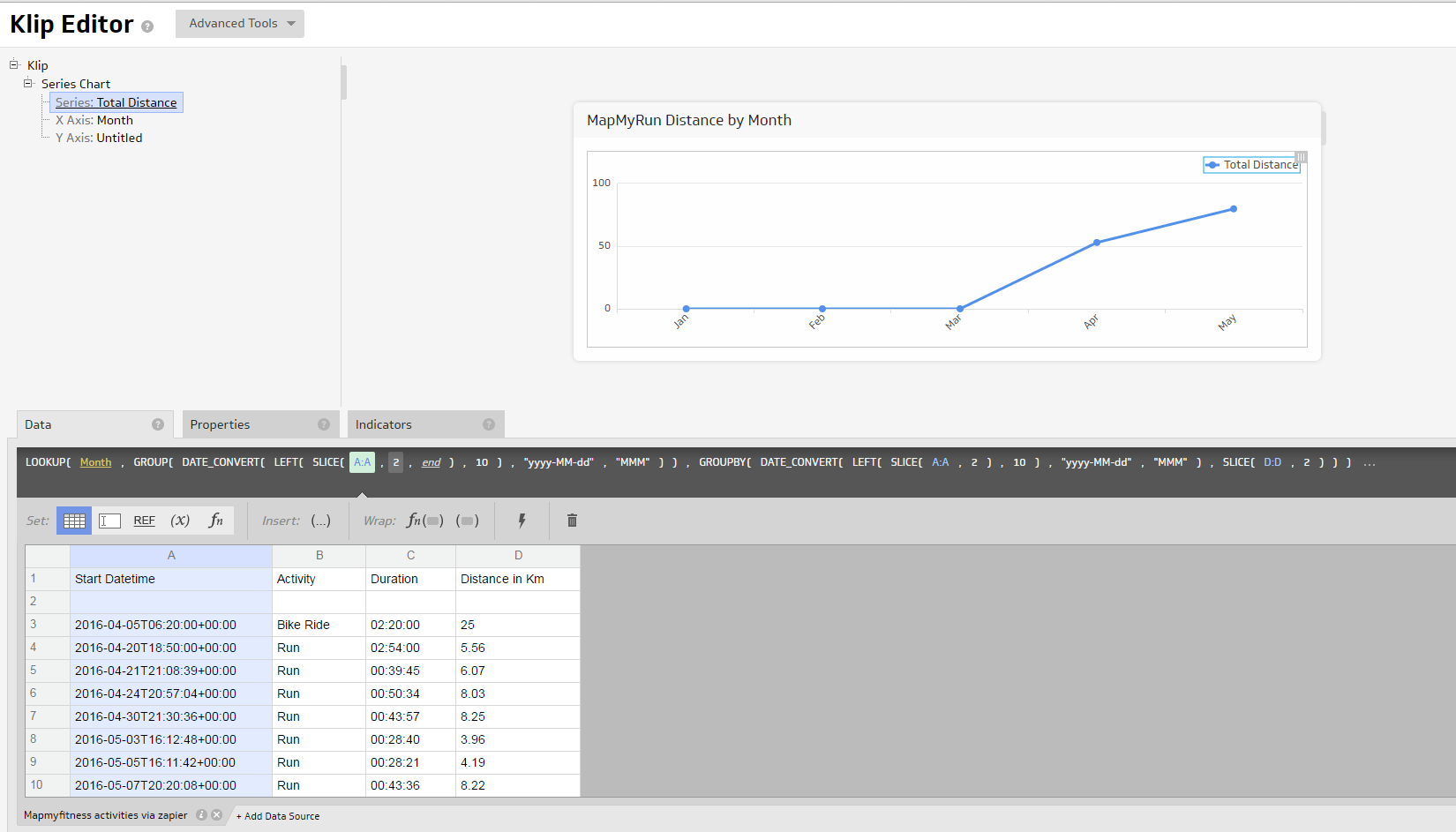References:

https://support.klipfolio.com/hc/en-us/articles/215547998-GROUP-and-GROUPBY-functions-?query=Group

https://support.klipfolio.com/hc/en-us/articles/216182777-LOOKUP-function-?query=LookUp

https://support.klipfolio.com/hc/en-us/articles/215547648-DATE-CONVERT-function-?query=date_convert

~Kavya Mallur

•T V

Kavya -

Thanks for ^that^. It was exactly what I was looking to do as well!

--Trudy

•Iris Ten Teije

I want to do something similar, but imagine instead of adding up the kilometres in column D, I want to simply count the amount of times I did a run / bike ride in that month. How should I write the function? (so the outcome would be, April -> 5).

•Janice Janczyn

Hi Iris,

To count the number of times an item occurs within a grouping, use the COUNTDISTINCT function (described here) instead of GROUPBY. Note that I'm using a different approach to set the display format of the x-axis compared with the original solution and then using a results reference in the LOOKUP instead of a formula reference which returns the Display format instead of the Input format of the Months in the x-axis.

Month x-axis

Data (formula):  GROUP(DATEVALUE(DATERANGE(DATE_STARTOF(TODAY(), year), TODAY()),"yyyyMM"))
Properties:  set Format to    Date/Time
Input     to    YYYYMM
Display to    MMM yyyy (select Aug 2016)

Run series

LOOKUP( &X Axis: Month,
GROUP( SELECT( DATE_CONVERT( @A:A, "yyyy-MM-dd", "MMM yyyy"), @B:B = "Run")),
COUNTDISTINCT( SELECT( DATE_CONVERT( @A:A, "yyyy-MM-dd", "MMM yyyy"), @B:B = "Run") ) ) )

Thanks,
Janice

•Junior Vanden Brink

It seems that creating bar charts and line graphs that group dates by Month is a really really common necessity in basic dash-boarding. Is there a way to do this without an 8 layer deep nested formula like explained above?

•Janice Janczyn

Hi Junior,

With PowerMetrics you can quickly and easily change the time periods (day/week/month) and timeframes displayed by your visualizations. What are PowerMetrics gives a video overview of PowerMetrics and Introduction to PowerMetrics describes the functionality and has links to other PowerMetrics articles.

Thanks,
Janice